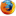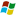# wordpress使用jQuery调整图片宽高度

• 正文内容
• 相关推荐

jQuery调整图片宽高

```\$(document).ready(function() {
\$('.post img').each(function() {
var maxWidth = 600; // 图片最大宽度
var maxHeight = 2000;    // 图片最大高度
var ratio = 0;  // 缩放比例
var width = \$(this).width();    // 图片实际宽度
var height = \$(this).height();  // 图片实际高度

// 检查图片是否超宽
if(width > maxWidth){
ratio = maxWidth / width;   // 计算缩放比例
\$(this).css("width", maxWidth); // 设定实际显示宽度
height = height * ratio;    // 计算等比例缩放后的高度
\$(this).css("height", height * ratio);  // 设定等比例缩放后的高度
}

// 检查图片是否超高
if(height > maxHeight){
ratio = maxHeight / height; // 计算缩放比例
\$(this).css("height", maxHeight);   // 设定实际显示高度
width = width * ratio;    // 计算等比例缩放后的高度
\$(this).css("width", width * ratio);    // 设定等比例缩放后的高度
}
});
});```

```<script type="text/javascript">
function ResizeImage(image, 插图最大宽度, 插图最大高度)
{
if (image.className == "Thumbnail")
{
w = image.width;
h = image.height;

if( w == 0 || h == 0 )
{
image.width = maxwidth;
image.height = maxheight;
}
else if (w > h)
{
if (w > maxwidth) image.width = maxwidth;
}
else
{
if (h > maxheight) image.height = maxheight;
}

image.className = "ScaledThumbnail";
}
}
</script>```### 评论

1条评论
1.Firefox 56.0Windows 回复

反复拜读了，理清了很多。

广东省深圳市福田区 电信

• 评论

#### 大家都在重庆seo博客搜